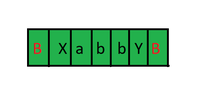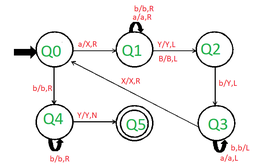# Construct Turing Machine for L = {a^i b^j | i<j, i>0}

• Last Updated : 23 Sep, 2020

Prerequisite – Turing Machine

We have to design a Turing machine for a^i b^j where i<j and i>0.

Analysis :
Here the main thing to notice that i<j. It means that the count of ‘b’ in the string is always greater than the count of ‘a’. Therefore we can write a^i b^j like that –

`a^i b^j = a^n b^n + extra number of b.`

Examples –

```Input: aabbbb
Output: Accepted

Input: aaabb
Output: Not Accepted```

Approach :
Let us understand the approach by taking a string “aabbb”.

1. Scan the input from the left.
2. First, replace an ‘a’ with ‘X’ and move 1 step right. Then skip all the a’s and b’s and move right.
3. When the pointer reaches Blank(B) it scans the input from the right and replaces the first ‘b’ with ‘Y’.4. Again the pointer reaches Blank(B). It now scans the input from left to right. The pointer moves forward and replaces ‘a’ with ‘X’.
5. Again the pointer reaches Blank(B). It now scans the input from the right to left. The pointer moves forward and replaces ‘b’ with ‘y’.
6. We repeat the same steps until we convert all the a’s to ‘X’. b’s equal to the count of a’s will also convert to ‘Y’ and we will be left with some of the remaining b’s.My Personal Notes arrow_drop_up